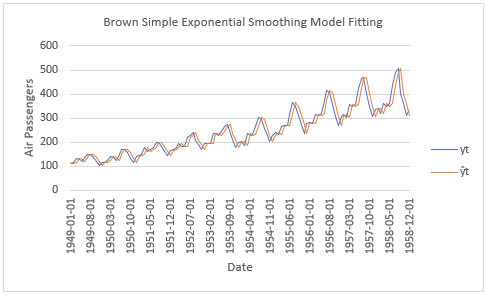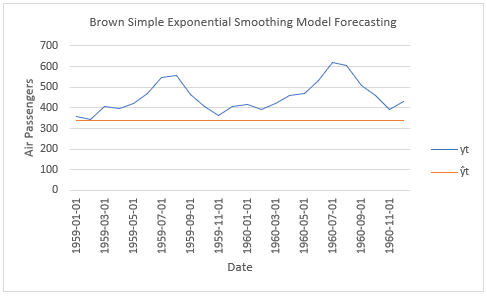# Exponential Smoothing: Brown Simple Method

Last Update: April 24, 2022

Exponential Smoothing is a forecasting method which flattens time series data. Brown Simple Exponential Smoothing Method  is used for forecasting time series data with no trend or seasonal patterns. It has an ETS(A,N,N) notation with additive errors and no trend or seasonal components.

As example, we can delimit univariate time series  into training range  for model fitting and testing range  for model forecasting.

Then, we can fit model using Brown simple exponential smoothing method with formula . Training range model fitted values  are the one step ahead estimated  values and  is the level component with formula . Training range level component fitted values  are the estimated  values and  is the level smoothing coefficient.

Training range model fitting can be done by estimating optimal level smoothing coefficient  and optimal initial level fitted value  through minimizing sum of squared estimated model residuals with formula . Training range estimated model residuals  with formula  are the differences between actual  and fitted  values. Notice that initial level fitted value can also be estimated using simple formula .

Next, we can forecast model using Brown simple exponential smoothing method with formula . Testing range model forecasted values  are the  steps ahead estimated  values and  is the last training range level component fitted value with formula . Notice it is important to remember that when doing time series analysis and forecasting, past performance does not guarantee future results.

Below, we find example of model fitting and forecasting using Brown simple exponential smoothing method for airline passengers with training range as first ten years and testing range as last two years of data . Optimal level smoothing coefficient and optimal initial level fitted value estimations done using Microsoft Excel® Solver® Add-in.Figure 1. Microsoft Excel® model fitting using Brown simple exponential smoothing method for airline passengers with training range as first ten years and testing range as last two years of data.Figure 2. Microsoft Excel® model forecasting using Brown simple exponential smoothing method for airline passengers with training range as first ten years and testing range as last two years of data.

 Brown, Robert G. (1956). “Exponential Smoothing for Predicting Demand”. Cambridge, Massachusetts: Arthur D. Little Inc. p. 15.

 Hyndman, R.J., Koehler, A.B., Ord, J.K., Snyder, R.D. (2008). “Forecasting with exponential smoothing: the state space approach”, Springer-Verlag: New York.

 Data Description: Monthly international airline passenger numbers in thousands from 1949 to 1960.

Original Source: Box, G. E. P., Jenkins, G. M. and Reinsel, G. C. (1976). “Time Series Analysis, Forecasting and Control”. Third Edition. Holden-Day. Series G.

Source: datasets R Package AirPassengers Object. R Core Team (2021). “R: A language and environment for statistical computing”. R Foundation for Statistical Computing, Vienna, Austria.

+